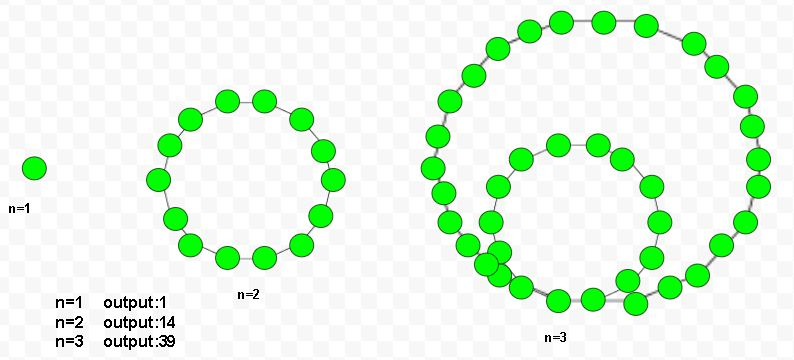Given a number n, the task is to find the nth tetradecagonal number. A tetradecagonal number is 14-sided polygon called tetrakaidecagon or tetradecagon and belongs to the figurative number. The nth tetradecagonal number doted with some dots and create a series of the pattern. They have a common sharing corner point and doted with their spaces to each other. The dots continue with nth nested loop.

Examples :

Input : 5
Output :125

Input :7
Output :259Formula for nth tetradecagonal number :-## C++

 // Program to find nth  // Tetradecagonal number  #include  using namespace std;     // Function to find  // Tetradecagonal number  int tetradecagonal_num(int n)  {      // Formula to calculate nth      // tetradecagonal number=      return (12 * n * n - 10 * n) / 2;  }     // Driver Code  int main()  {      int n = 2;      cout << n << " th Tetradecagonal number: ";      cout << tetradecagonal_num(n);      cout << endl;      n = 6;      cout << n << " th Tetradecagonal number: ";      cout << tetradecagonal_num(n);         return 0;  }

## Java

 // Java Program to find nth  // Tetradecagonal number  import java.io.*;     class GFG   {         // Function to find  // Tetradecagonal number  static int tetradecagonal_num(int n)  {      // Formula to calculate nth      // tetradecagonal number=      return (12 * n * n - 10 * n) / 2;  }     // Driver Code  public static void main (String[] args)   {  int n = 2;  System.out.print(n + " th Tetradecagonal" +                                " number: ");  System.out.println(tetradecagonal_num(n));     n = 6;  System.out.print(n + " th Tetradecagonal" +                                " number: ");  System.out.print(tetradecagonal_num(n));     }  }  // This code is code is  // contributed by m_kit

## Python 3

 # Program to find nth  # Tetradecagonal number     # Tetradecagonal number  # number function  def tetradecagonal_num(n) :             # Formula to calculate      # nth Tetradecagonal       # number return it       # into main function.      return (12 * n * n -              10 * n) // 2    # Driver Code  if __name__ == '__main__' :                 n = 2     print(n,"th Tetradecagonal " +                      "number : " ,               tetradecagonal_num(n))         n = 6     print(n,"th Tetradecagonal " +                      "number : " ,               tetradecagonal_num(n))     # This code is contributed ajit

## C#

 // C# Program to find nth  // Tetradecagonal number  using System;     class GFG  {             // Function to find      // Tetradecagonal number      static int tetradecagonal_num(int n)      {                 // Formula to calculate nth      // tetradecagonal number      return (12 * n * n -               10 * n) / 2;      }     // Driver Code  static public void Main ()  {      int n = 2;      Console.Write(n + "th Tetradecagonal" +                                " number: ");      Console.WriteLine(tetradecagonal_num(n));         n = 6;      Console.Write(n + "th Tetradecagonal" +                                " number: ");      Console.WriteLine(tetradecagonal_num(n));  }  }     // This code is contributed by ajit

## PHP

 

Output :

2 th Tetradecagonal number: 14


Attention reader! Don’t stop learning now. Get hold of all the important DSA concepts with the DSA Self Paced Course at a student-friendly price and become industry ready.

My Personal Notes arrow_drop_upCheck out this Author's contributed articles.

If you like GeeksforGeeks and would like to contribute, you can also write an article using contribute.geeksforgeeks.org or mail your article to contribute@geeksforgeeks.org. See your article appearing on the GeeksforGeeks main page and help other Geeks.

Please Improve this article if you find anything incorrect by clicking on the "Improve Article" button below.

Improved By : jit_t